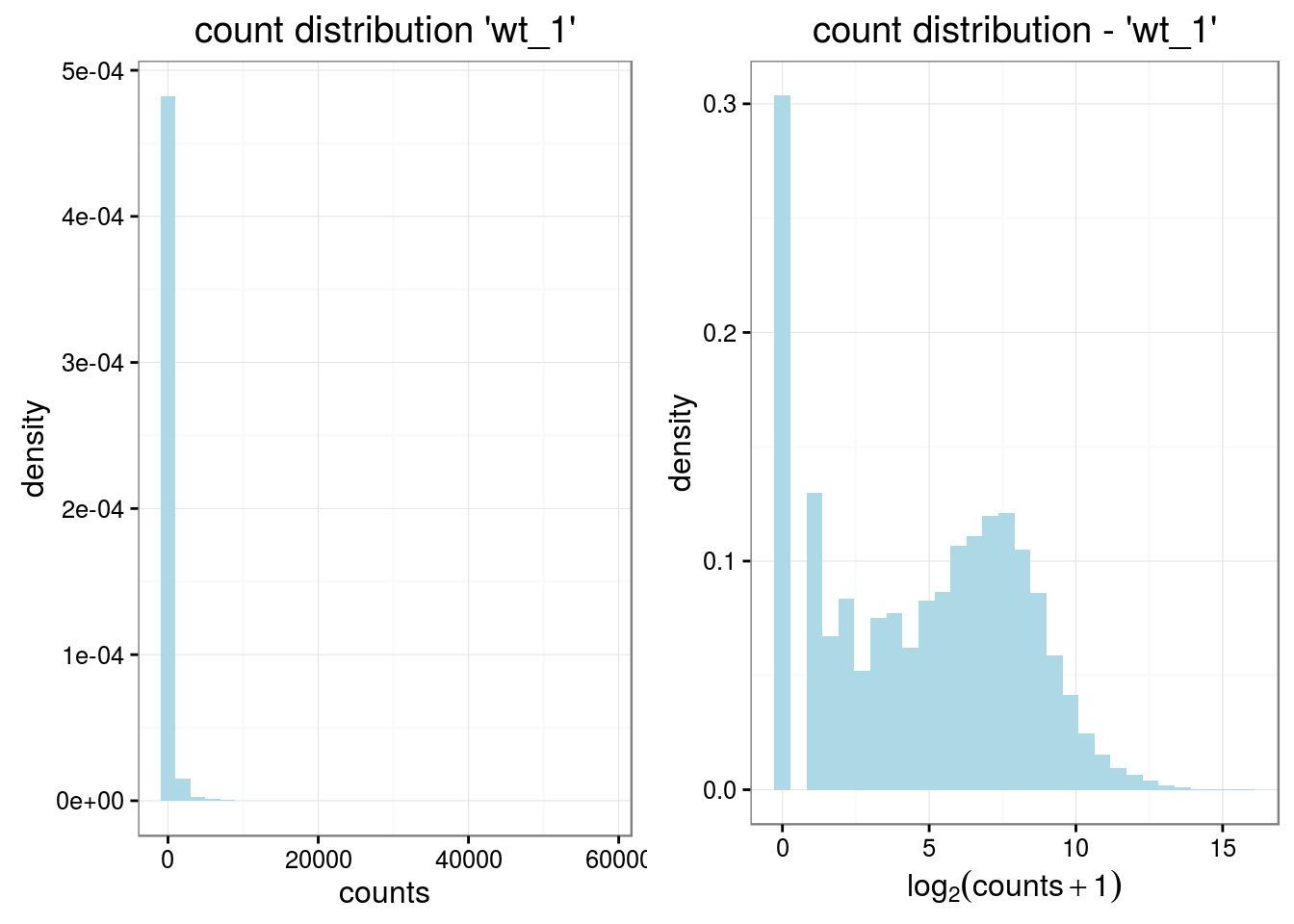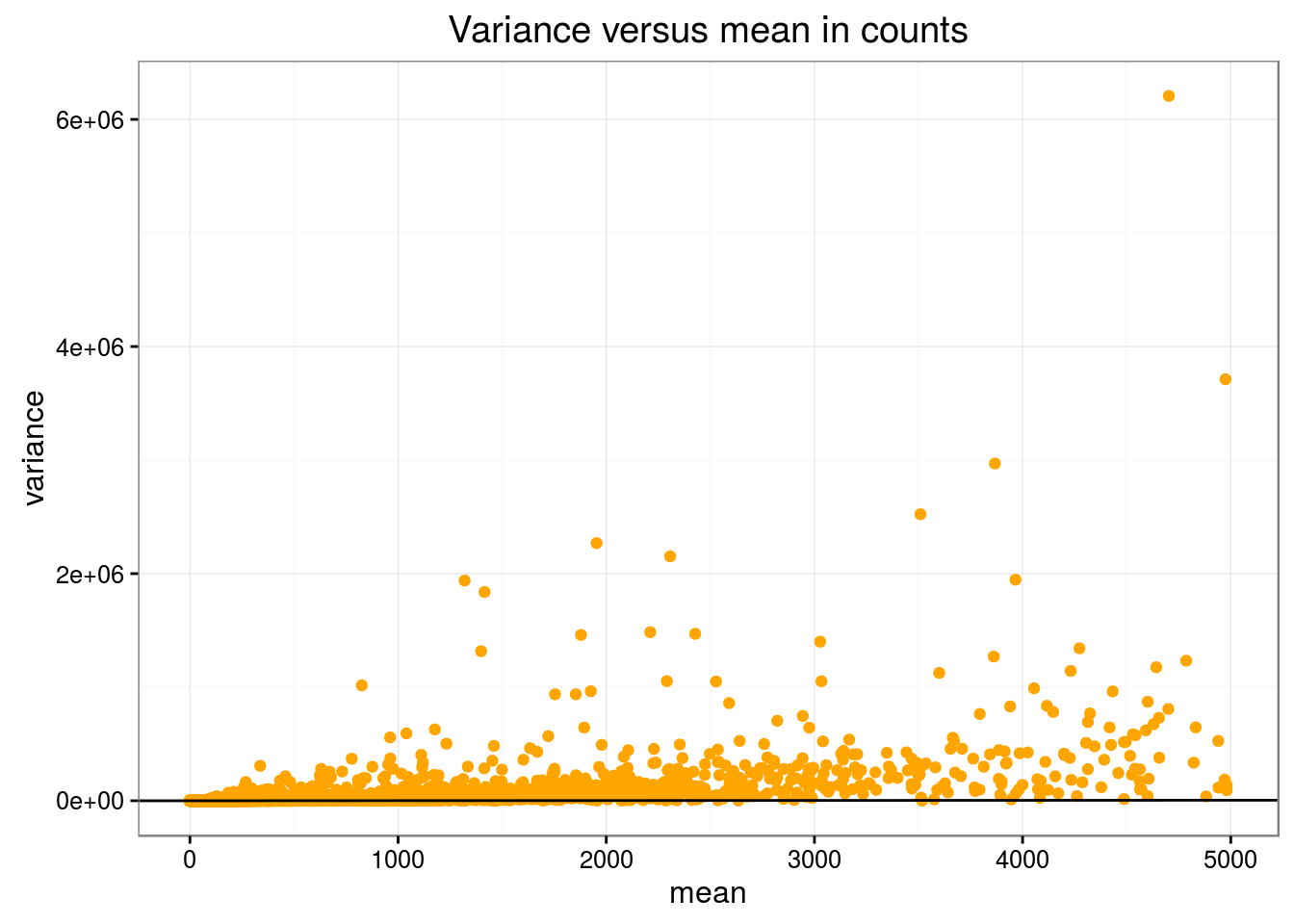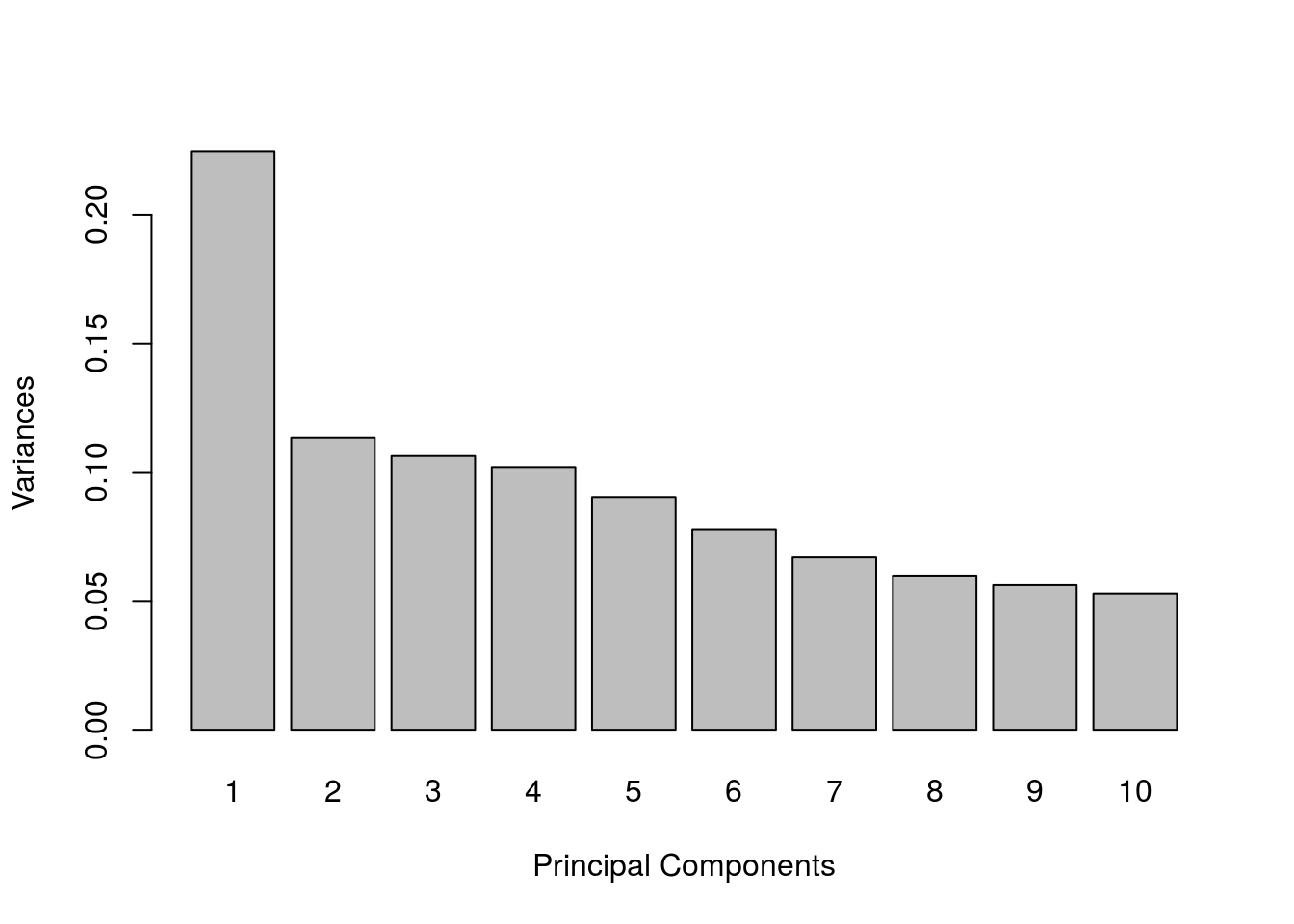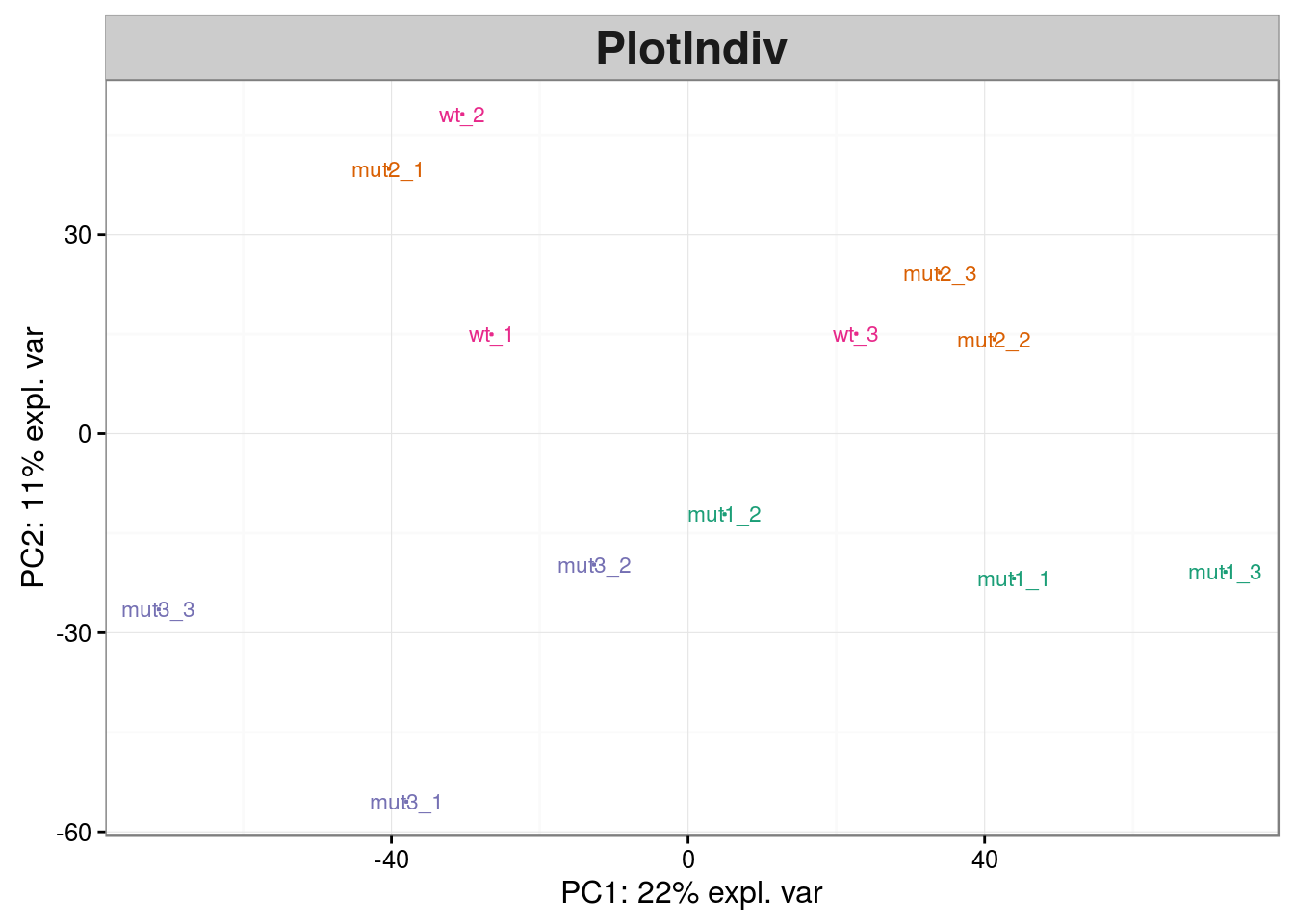This file illustrates the normalization and differential analysis of RNAseq data on a real life dataset. The data are provided by courtesy of the transcriptomic platform of IPS2 and the name of the genes were shuffled. Hence the results are not biologically interpretable. They are composed of three files, all included in the directory data:

• D1-counts.txt contains the raw counts of the experiment (13 columns: the first one contains the gene names, the others correspond to 12 different samples);

• D1-genesLength.txt contains information about gene lengths;

• D1-targets.txt contains information about the sample and the experimental design.

In the rest of this file, we:

1/ first import and describe the data;

2/ then perform and compare different normalizations;

3/ finally perform a differential analysis using different methods and models.

We advice that you use the file by reading the R code while trying to make sense of it. Then, you run it and analyze the results. The packages devtools, ggplot2, gridExtra, reshape2, mixOmics, RColorBrewer, DESeq, edgeR and VennDiagram are required to compile this file. Information about my system (including package and R versions) are provided at the end of this file, in Section “Session information”.

library(ggplot2)
library(gridExtra)
library(reshape2)
library(mixOmics)
library(RColorBrewer)
library(DESeq)
library(edgeR)
library(VennDiagram)
library(devtools)

## Data description and importation

The data used in this practical session correspond to 12 samples of RNAseq obtained from microdissections on plants. Plants have four different genotypes: the wild type (“wt”“) plant and three types of mutants and are observed three times in the same conditions. for information Mutants 1 and 2 have a similar phenotype (more complicated leaves than the wild type) whereas mutant 3 has the opposite phenotype (simpler leaves than the wild type).

The datasets are included in the repository data located at the root of the material for this practical session. They can be loaded using:

raw_counts <- read.table("../data/D1-counts.txt", header = TRUE, row.names = 1)
raw_counts <- as.matrix(raw_counts)
stringsAsFactors = FALSE)
gene_lengths <- scan("../data/D1-genesLength.txt")

### Raw counts

The dimensions of the raw count matrix are equal to:

dim(raw_counts)
##  50897    12

which shows that the data contains 12 columns (samples) and 50897 rows (genes). And a quick look is obtained by:

head(raw_counts)
##                  wt_1 wt_2 wt_3 mut1_1 mut1_2 mut1_3 mut2_1 mut2_2 mut2_3
## Medtr0001s0010.1    0    0    0      1      0      1      0      0      0
## Medtr0001s0070.1    0    0    0      0      0      0      0      0      0
## Medtr0001s0100.1    0    0    0      0      0      0      0      0      0
## Medtr0001s0120.1    0    0    0      0      0      0      0      0      0
## Medtr0001s0160.1    0    0    0      0      0      0      0      0      0
## Medtr0001s0190.1    0    0    0      0      0      0      0      0      0
##                  mut3_1 mut3_2 mut3_3
## Medtr0001s0010.1      0      1      0
## Medtr0001s0070.1      0      0      0
## Medtr0001s0100.1      0      0      0
## Medtr0001s0120.1      0      0      0
## Medtr0001s0160.1      0      0      0
## Medtr0001s0190.1      0      0      0

which shows that the row names are gene names and that the data are made of counts. Many of these counts are equal to 0. A basic exploratory analysis of the count data is provided in the next section.

### Experimental design

The experimental design is described in the object design:

design
##    labels group replicat
## 1    wt_1    wt  repbio1
## 2    wt_2    wt  repbio2
## 3    wt_3    wt  repbio3
## 4  mut1_1  mut1  repbio1
## 5  mut1_2  mut1  repbio2
## 6  mut1_3  mut1  repbio3
## 7  mut2_1  mut2  repbio1
## 8  mut2_2  mut2  repbio2
## 9  mut2_3  mut2  repbio3
## 10 mut3_1  mut3  repbio1
## 11 mut3_2  mut3  repbio2
## 12 mut3_3  mut3  repbio3

that contains 3 columns: the first one labels is the sample name, the second one group is the group of the sample (wild type or one of three types of mutant) and the last one replicat is the biological replicate number.

## Basic exploratory analysis of raw counts

We start the analysis by filtering out the genes for which no count has been found:

raw_counts_wn <- raw_counts[rowSums(raw_counts) > 0, ]
dim(raw_counts_wn)
##  27916    12

The number of genes which have been filtered out is thus equal to 22981.

It is often useful, to visualize the count distribution, to compute “pseudo counts”, which are log-transformed counts:

pseudo_counts <- log2(raw_counts_wn + 1)
head(pseudo_counts)
##                      wt_1     wt_2     wt_3   mut1_1   mut1_2   mut1_3
## Medtr0001s0010.1 0.000000 0.000000 0.000000 1.000000 0.000000 1.000000
## Medtr0001s0200.1 0.000000 1.000000 0.000000 0.000000 0.000000 0.000000
## Medtr0001s0260.1 6.357552 6.768184 6.643856 6.169925 6.507795 6.189825
## Medtr0001s0360.1 0.000000 0.000000 1.000000 0.000000 0.000000 0.000000
## Medtr0001s0490.1 1.000000 2.000000 0.000000 0.000000 0.000000 0.000000
## Medtr0002s0040.1 7.707359 6.918863 7.826548 2.584963 2.000000 4.643856
##                    mut2_1   mut2_2   mut2_3   mut3_1   mut3_2   mut3_3
## Medtr0001s0010.1 0.000000 0.000000 0.000000 0.000000 1.000000 0.000000
## Medtr0001s0200.1 0.000000 0.000000 0.000000 0.000000 0.000000 0.000000
## Medtr0001s0260.1 6.209453 6.392317 6.672425 6.820179 6.554589 6.539159
## Medtr0001s0360.1 0.000000 1.584963 0.000000 1.000000 0.000000 0.000000
## Medtr0001s0490.1 0.000000 0.000000 1.584963 1.000000 0.000000 1.000000
## Medtr0002s0040.1 6.643856 7.651052 8.044394 3.169925 3.459432 1.584963
df_raw <- melt(pseudo_counts, id = rownames(raw_counts_wn))
names(df_raw)[1:2]<- c("id", "sample")
df_raw$method <- rep("Raw counts", nrow(df_raw)) head(df_raw) ## id sample value method ## 1 Medtr0001s0010.1 wt_1 0.000000 Raw counts ## 2 Medtr0001s0200.1 wt_1 0.000000 Raw counts ## 3 Medtr0001s0260.1 wt_1 6.357552 Raw counts ## 4 Medtr0001s0360.1 wt_1 0.000000 Raw counts ## 5 Medtr0001s0490.1 wt_1 1.000000 Raw counts ## 6 Medtr0002s0040.1 wt_1 7.707359 Raw counts The object df_raw will be used later to compare the effect of different normalization on the count distribution in the different samples. ### Count distribution First, we provide an overview of the distribution of the first sample (without the genes that have been filtered out) by ploting the histograms of raw counts and pseudo counts: df <- data.frame(rcounts = raw_counts_wn[ ,1], prcounts = pseudo_counts[ ,1]) p <- ggplot(data=df, aes(x = rcounts, y = ..density..)) p <- p + geom_histogram(fill = "lightblue") p <- p + theme_bw() p <- p + ggtitle(paste0("count distribution '", design$labels, "'"))
p <- p + xlab("counts")

p2 <- ggplot(data=df, aes(x = prcounts, y = ..density..))
p2 <- p2 + geom_histogram(fill = "lightblue")
p2 <- p2 + theme_bw()
p2 <- p2 + ggtitle(paste0("count distribution - '", design$labels, "'")) p2 <- p2 + xlab(expression(log(counts + 1))) grid.arrange(p, p2, ncol = 2)This figure shows that the count distribution is highly skewed with a large proportion of genes with a count equal to 0 and a few number of genes with an extreme count (more that $$2^{15}$$). ### Relation between mean and variance Another important feature of RNAseq data is the fact that they are overdispersed. This means that the variance of the count of a given gene over different biological samples within a given condition is larger than the average count for the same gene. This feature is illustrated by plotting the graphics of the mean vs variance for condition “wt” for all genes with an average count smaller than 5000 (otherwise the chart can not be read easily): df <- data.frame(mean = apply(raw_counts_wn[ ,design$group == "wt"], 1, mean),
var = apply(raw_counts_wn[ ,design$group == "wt"], 1, var)) df <- df[df$mean <= 5000, ]
p <- ggplot(data=df, aes(x = mean, y = var))
p <- p + geom_point(colour = "orange")
p <- p + theme_bw()
p <- p + geom_abline(aes(intercept=0, slope=1))
p <- p + ggtitle("Variance versus mean in counts") + ylab("variance")
print(p)In this figure, the black line is the “y=x” diagonal. It is easy to see that, for most genes, the variance is much larger than the mean.

### PCA

Finally, a PCA is performed to identify the main sources of variability in the dataset. Pseudo-counts are used to perform this PCA.

resPCA <- pca(t(pseudo_counts), ncomp = 12)
plot(resPCA)The first component explains more variance than the other components which almost all equally reproduce variance. The projection of the samples on the first two PCs is obtained with:

plotIndiv(resPCA, group = design$group, col.per.group = brewer.pal(4, "Dark2"))This figure shows that mutants 1 and 3 are well separated from the other wild type but that wild type is not well separated from mutant 2. ## Normalization This section performs different normalization approaches for RNAseq data. These normalization are performed using either DESeq (RLE) or edgeR (TC, RPKM, UQ, TMM). The final count distribution is compared to the raw count distribution in the last part of this section. ### DESeq To perform the normalization with DESeq, an object of type CountDataSet has to be created: dge <- newCountDataSet(raw_counts_wn, conditions = design$group)
dge
## CountDataSet (storageMode: environment)
## assayData: 27916 features, 12 samples
##   element names: counts
## protocolData: none
## phenoData
##   sampleNames: wt_1 wt_2 ... mut3_3 (12 total)
##   varLabels: sizeFactor condition
## featureData: none
## experimentData: use 'experimentData(object)'
## Annotation:

In this object, the attribute sizeFactor is at first missing:

sizeFactors(dge)
##   wt_1   wt_2   wt_3 mut1_1 mut1_2 mut1_3 mut2_1 mut2_2 mut2_3 mut3_1
##     NA     NA     NA     NA     NA     NA     NA     NA     NA     NA
## mut3_2 mut3_3
##     NA     NA

The function estimateSizeFactors() allows to estimate the size factors. The dge object is unchanged but the attribute sizeFactor is now given:

dge <- estimateSizeFactors(dge)
sizeFactors(dge)
##      wt_1      wt_2      wt_3    mut1_1    mut1_2    mut1_3    mut2_1
## 0.9753728 1.1491346 1.0187414 0.9167701 1.0326086 1.1436435 0.9664323
##    mut2_2    mut2_3    mut3_1    mut3_2    mut3_3
## 0.9570126 0.9826726 1.1355102 0.9271699 0.9202688

Normalized counts can be obtained using the function counts with the option normalized = TRUE. The calculation of the normalized counts is performed with the formula $$\tilde{K}_{gj} = \frac{K_{gj}}{s_j}$$. The following command lines compare the result of the counts function with this calculation:

deseq_normcount <- counts(dge, normalized = TRUE)
test_normcount <- sweep(raw_counts_wn, 2, sizeFactors(dge), "/")
sum(test_normcount != deseq_normcount)
##  0

Finally, pseudo counts are computed and stored in a data frame for further comparison.

pseudo_deseq <- log2(deseq_normcount + 1)
df_deseq <- melt(pseudo_deseq, id = rownames(raw_counts_wn))
names(df_deseq)[1:2]<- c("id", "sample")
df_deseq$method <- rep("DESeq (RLE)", nrow(df_raw))  ### edgeR In this section, the package edgeR is perform to compare the other normalization approaches. To do so, a DGEList object has to be created from the count data: dge2 <- DGEList(raw_counts_wn) dge2 ## An object of class "DGEList" ##$counts
##                  wt_1 wt_2 wt_3 mut1_1 mut1_2 mut1_3 mut2_1 mut2_2 mut2_3
## Medtr0001s0010.1    0    0    0      1      0      1      0      0      0
## Medtr0001s0200.1    0    1    0      0      0      0      0      0      0
## Medtr0001s0260.1   81  108   99     71     90     72     73     83    101
## Medtr0001s0360.1    0    0    1      0      0      0      0      2      0
## Medtr0001s0490.1    1    3    0      0      0      0      0      0      2
##                  mut3_1 mut3_2 mut3_3
## Medtr0001s0010.1      0      1      0
## Medtr0001s0200.1      0      0      0
## Medtr0001s0260.1    112     93     92
## Medtr0001s0360.1      1      0      0
## Medtr0001s0490.1      1      0      1
## 27911 more rows ...
##
## $samples ## group lib.size norm.factors ## wt_1 1 5932414 1 ## wt_2 1 7129204 1 ## wt_3 1 6223759 1 ## mut1_1 1 5577595 1 ## mut1_2 1 6272344 1 ## 7 more rows ... The library sizes and the normalization factors for all samples are obtained by: dge2$samples
##        group lib.size norm.factors
## wt_1       1  5932414            1
## wt_2       1  7129204            1
## wt_3       1  6223759            1
## mut1_1     1  5577595            1
## mut1_2     1  6272344            1
## mut1_3     1  6634542            1
## mut2_1     1  5897356            1
## mut2_2     1  5785184            1
## mut2_3     1  5767730            1
## mut3_1     1  7106881            1
## mut3_2     1  5872052            1
## mut3_3     1  6035692            1

#### Total count

Normalization by Total Count (TC) is obtained directly by the function cpm. Pseudo-counts ($$\log_2$$ transformed counts) are computed and stored in a data frame for later use.

pseudo_TC <- log2(cpm(dge2) + 1)

df_TC <- melt(pseudo_TC, id = rownames(raw_counts_wn))
names(df_TC)[1:2] <- c ("id", "sample")

#### Upper quartile

Upper quartile normalization is obtained with the function calcNormFactors. This function changes the variable norm.factors in the DGEList object and thus also the way the functions cpm and rpkm are computing counts: now, a normalized library size, equal to the original library size multiplied by the normalization factor, is used to compute normalized counts: $$\tilde{K}_{gj} = \frac{K_{gj}}{\mbox{modified LS}}*10^6$$. The normalization factors are computed using:

dge2 <- calcNormFactors(dge2, method = "upperquartile")
dge2$samples ## group lib.size norm.factors ## wt_1 1 5932414 1.0047308 ## wt_2 1 7129204 0.9810476 ## wt_3 1 6223759 1.0130558 ## mut1_1 1 5577595 1.0068753 ## mut1_2 1 6272344 0.9942229 ## mut1_3 1 6634542 1.0593861 ## mut2_1 1 5897356 0.9873347 ## mut2_2 1 5785184 1.0124342 ## mut2_3 1 5767730 1.0453656 ## mut3_1 1 7106881 0.9792811 ## mut3_2 1 5872052 0.9857220 ## mut3_3 1 6035692 0.9361638 The previous formula is compared to the result of the function cpm in the following command lines: test_normcount <- sweep(dge2$counts, 2,
dge2$samples$lib.size*dge2$samples$norm.factors / 10^6,
"/")
range(as.vector(test_normcount - cpm(dge2)))
##  -1.818989e-12  0.000000e+00

which shows no difference. Finally, pseudo counts are obtained and stored in a data frame:

pseudo_UQ <- log2(cpm(dge2) + 1)

df_UQ <- melt(pseudo_UQ, id = rownames(raw_counts_wn))
names(df_UQ)[1:2] <- c ("id", "sample")
df_UQ$method <- rep("UQ", nrow(df_UQ)) #### TMM TMM normalization works similarly as UQ and updates the value of the variable norm.factors: dge2 <- calcNormFactors(dge2, method = "TMM") dge2$samples
##        group lib.size norm.factors
## wt_1       1  5932414    1.0082044
## wt_2       1  7129204    0.9850930
## wt_3       1  6223759    1.0047944
## mut1_1     1  5577595    1.0064979
## mut1_2     1  6272344    1.0094894
## mut1_3     1  6634542    1.0593265
## mut2_1     1  5897356    0.9977013
## mut2_2     1  5785184    1.0096719
## mut2_3     1  5767730    1.0387295
## mut3_1     1  7106881    0.9791254
## mut3_2     1  5872052    0.9637238
## mut3_3     1  6035692    0.9429276

Normalized pseudo counts are obtained with the function cpm and stored in a data frame:

pseudo_TMM <- log2(cpm(dge2) + 1)

df_TMM <- melt(pseudo_TMM, id = rownames(raw_counts_wn))
names(df_TMM)[1:2] <- c ("id", "sample")
df_TMM$method <- rep("TMM", nrow(df_TMM)) ### Comparison This last section shows the comparison between all normalization methods (and original raw data) for this data set. First, the distributions of all samples and all normalization methods are compared with boxplots and density plots: df_allnorm <- rbind(df_raw, df_deseq, df_TC, df_RPKM, df_UQ, df_TMM) df_allnorm$method <- factor(df_allnorm\$method,
levels = c("Raw counts", "DESeq (RLE)", "TC",
"RPKM", "TMM", "UQ"))

p <- ggplot(data=df_allnorm, aes(x=sample, y=value, fill=method))
p <- p + geom_boxplot()
p <- p + theme_bw()
p <- p + ggtitle("Boxplots of normalized pseudo counts\n
for all samples by normalization methods")
p <- p + facet_grid(. ~ method)
p <- p + ylab(expression(log ~ (normalized ~ count + 1))) + xlab("")
p <- p + theme(title = element_text(size=10), axis.text.x = element_blank(),
axis.ticks.x = element_blank())
print(p)